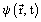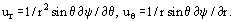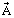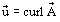# Stream Function

The stream function is a function of coordinates and timeand is a three-dimensional property of the hydrodynamics of an inviscid liquid, which allows us to determine the components of velocity by differentiating the stream function with respect to the given coordinates. A family of curves ψ = const represent "streamlines," hence, the stream function remains constant along a stream line.
In the case of a two-dimensional flow, the velocity components ux, uy are expressed in terms of the stream function with the help of formulas ux = ∂ψ/∂y, uy = −∂ψ/∂x. The difference ψ1 − ψ2 of the values of ψ for two streamlines can be interpreted as a volume, flow rate of a fluid in plane flow through a stream tube bounded by these two lines. In a potential plane flow the potential φ and the stream function ψ make a complex potential ω = φ + iψ, but the existence of the stream function is only related to the three-dimensional character of flow and in no way requires its potentiality. The stream function can be also defined for two-dimensional space flows, for instance, it is often used to describe a longitudinal flow past axisymmetrical bodies in a spherical system of coordinates, whereThe stream function represents a particular case of a vector potential of velocity, related to velocity by the equality. If there is a curvilinear system of coordinares in whichhas only one component, then it is exactly this system that represents the stream function for the given flow.